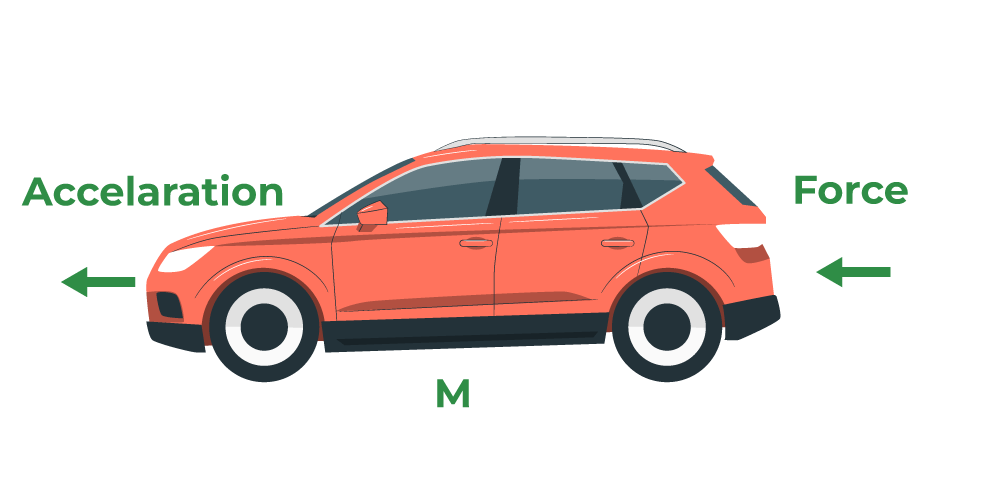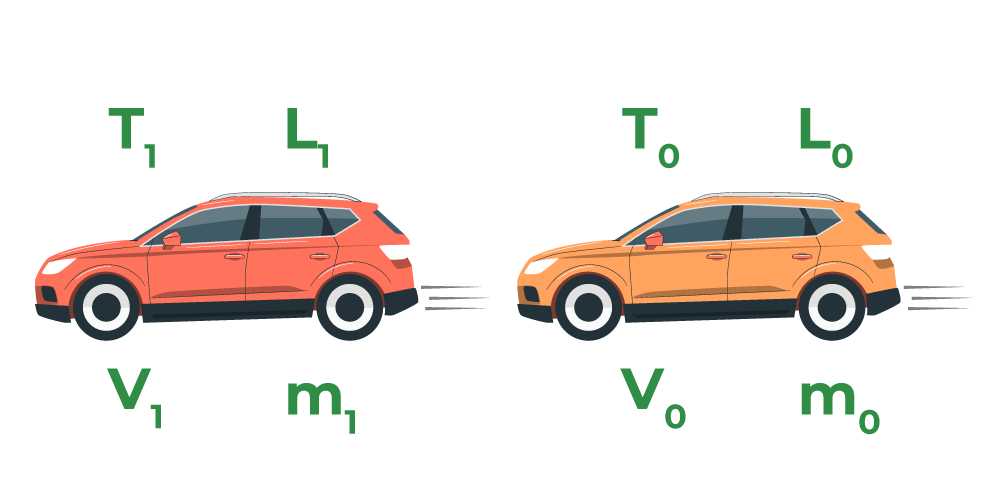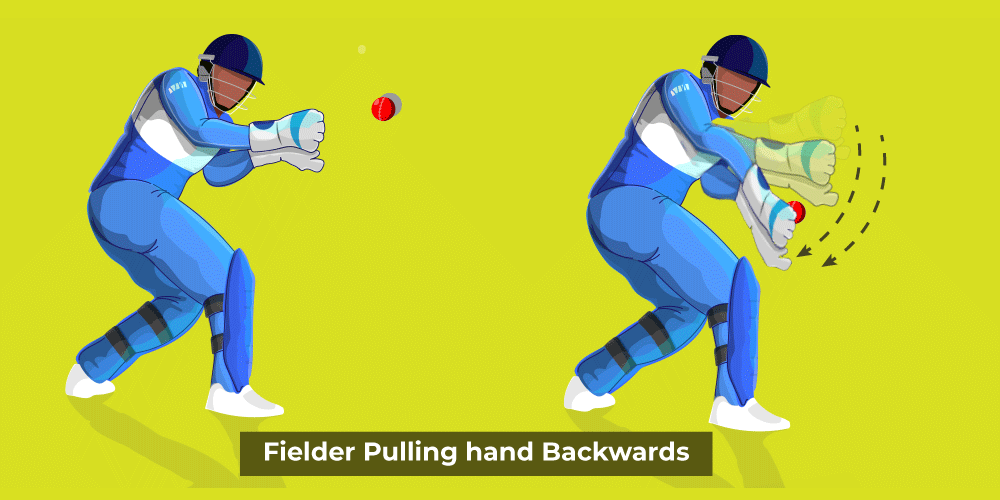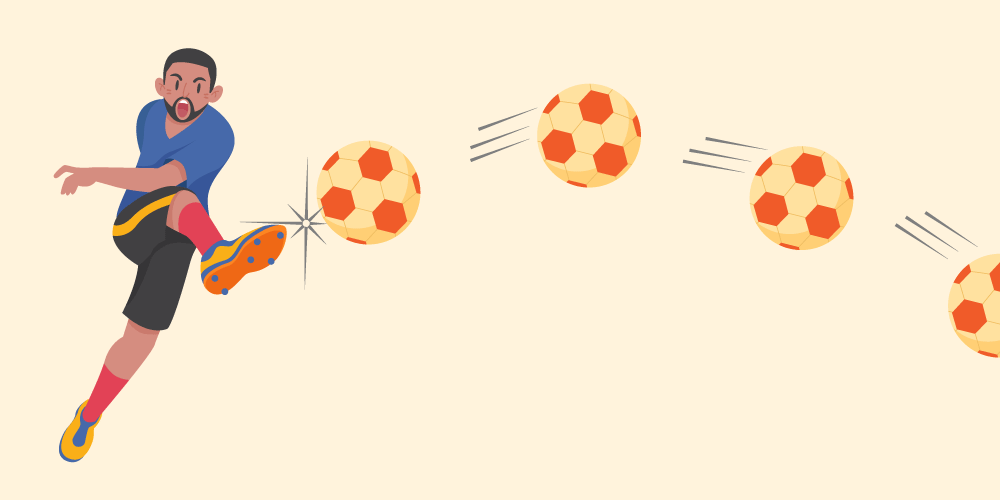GFG App
Open AppBrowser
Continue

# Newton’s Second Law of Motion

Newton’s Second Law of Motion tells us about the motion of objects experiencing unbalanced forces. It says that for an object under the influence of unbalanced forces, the acceleration of the object is directly proportional to the force applied. In other words, we can say that for a constant mass, the rate of change of momentum is directly proportional to the force applied. Now, in this article, we will learn about Newton’s Second Law of motion its example, and its application in detail.

## Newton’s Second Law of Motion

Sir Isaac Newton was the one who proposed the Law of Motion in the 17th century. The second law of motion provides a relationship between the force and acceleration of any object in the universe. It states that,

The rate of change of momentum of an object is proportional to the applied unbalanced force, in the direction of the force.

Mathematically, it is defined as,

Force ∝ Change in Momentum / Change in the Time

F ∝ dp / dt

where,
F is the Force
dp is the change in momentum
dt is the change in the time taken

## Derivation of Second law of Motion

The rate of change in the momentum of a body is directly proportional to the applied force and occurs in the force’s direction. Newton’s first law gives a realistic representation of force, whereas Newton’s second law gives a quantitative description of force.

Let us now consider a body with instantaneous velocity, and momentumwhich is given by,Since, according to the second law of motion,where,is the force acting on the object.

Also, since the momentum is defined as,Therefore, the previous equation becomes,where
k is the constant of proportionality

As the mass m of a body can be considered to be a constant quantity so derivative is applicable to the velocity of the body as shown below,It is known that the time rate change of velocity of the body is termed as its acceleration i.e.Therefore,The units of force are also chosen such that ‘k’ equals one.

As a result, if a unit force is selected to be the force causing a unit acceleration in a unit mass, i.e.,

F = 1 N, m = 1 kg and a = 1 ms-2. This implies, k = 1.

Thus, Newton’s second law of motion in mathematical form is given asThat is, the applied force of a body is defined as the product of its mass and acceleration. Hence, this provides us with a measure of the force.

If F = 0, we get a = 0.

This is similar to Newton’s first law of motion. That is, if there is no net external force, there will be no change in state of motion, implying that its acceleration is zero.

The image given below shows a car of mass ‘M’ accelerating at ‘a’ when the force applied is F.### For Variable Mass

Let us assume a body to be at an initial point (0) specified at location L0 and at the time instance t0. Let us assume the body has mass m0 and travels with a uniform velocity v0. On subjecting the body to a force F, the body moves to point 1, situated at the location L1 and reaches this point at time instance t1

The mass and velocity of the body undergo a transformation as the body travels to v1. Deriving the values for m1 and v1, we get,The image given below shows a car at two positions first at time T0 and the velocity at this time is V0 distance covered is L0 then at time T1 and the velocity at this time is V1 and the distance covered is L1.### For Constant Mass

For a constant mass, the usage of Newton’s second law can be equated as follows:## Momentum and Its Derivation

Momentum is the quantity of motion, which is multiplied by the amount of matter moved and the velocity at which it moves. When you walk, run, etc., you have momentum. If a bicycle and a car both drive down the street at the same speed, the automobile will have more momentum. Because velocity is considered a vector, momentum is also considered a vector.

The momentum of a body is the product of the mass of the body and its associated velocity. Momentum can be considered to be a vector quantity, that is, it has both an associated magnitude and direction.

Mathematically, the momentum (p) of an object of mass (m) moving with velocity (v) is defined as:

p= m × v

The unit of momentum is kg ms-1 and its dimensional formula is [MLT-1]

The second law of motion gives us a method to measure the force acting on an object as a product of its mass and the associated acceleration.

## Example of Newton’s Second Law of Motion

The second law of motion finds its usage in several domains:

• On the cricket ground, the fielder pulls his hands in the backward direction to catch the fast-approaching cricket ball. This reduces the momentum of the ball and induces a delay. When the ball comes into the hand of the fielder and comes to a halt, the momentum of the ball is reduced to zero. In case, the ball stops suddenly the momentum reaches 0 in an instant time frame. There is a quick rate of change in momentum due to which the player’s hand may get injured. Therefore, pulling the hand backwards a fielder induces a delay to the change of momentum to become zero, to prevent injury.

The image given below shows a fielder catching a ball and taking its hand backward to prevent injury.• A sand bed or cushioned bed is provided to athletes performing long jumps to induce a delay in the change of momentum. The momentum induced because of the velocity and mass of the athlete is reduced to zero as soon as the athlete reaches back on the surface. In case the rate of momentum changes instantly, it may hurt the player. The purpose of the cushioned bed is to delay the momentum of the athlete to zero and thus prevent injury.
• In case of a sudden halt to the vehicle, that is stoppage due to braking or accidents, the passenger tends to be pushed in the direction of motion of the vehicle and may get fatal injuries. In such a case, the change of momentum is reduced to zero. The stretchable seat belts increase the delay in the rate of momentum to be reduced to zero, to prevent injury.• An object falling down from a certain height undergoes an increase in acceleration because of the gravitational force applied.

## Application of Second Law

Newton’s second law finds its application in various types of examples that we encounter in our daily life. Let’s take a close look at some examples where force is applied according to Newton’s Second Law of Motion.

### Pushing an Object

We all have observed that pushing a lighter object is far easier than pushing a heavier object of similar characteristics. This observation comprehends Newton’s Second Law of Motion.

### Kicking a Football

Kicking a football changes its direction and also changes its velocity. It can increase or decrease the velocity of football. The force applied by the footballer is responsible for the change that the ball produces. Thus, Newton’s Second Law of Motion also holds true in this case.### Walking of Two People

If two people are walking their speed is directly dependent on their mass. In the event of applying similar force, the lighter one will have more speed compared to the lighter one confirming Newton’s Second Law of Motion.

### Acceleration of Rocket

Acceleration of the rocket is due to the force applied called Thrust. This force makes the rocket go up with an acceleration of ‘a’ where a is Thrust divided by mass.## Solved Examples on Newton’s Second Law of Motion

Example 1: If a bullet of mass 40 gm is shot from an Assault Riffle that has an initial velocity of 80 m/s the mass of the Assault Riffle is 15 kg. What is the initial recoil velocity of the Assault Riffle?

Solution:

Given,

Mass of bullet (m1) = 40 gm or 0.04 kg

Mass of the Assault Riffle (m2) = 15 kg

Initial velocity (v1) = 80 m/s.

Therefore, according to the law of conservation of momentum,

0 = 0.04 × 80 + 15 × v

15 × v = -3.2

v = -3.2 / 15

= -0.21 m/s

Example 2: If an object of mass 20 kg is moving with a constant velocity of 8 m/s on the frictionless ground. What will be the force required to keep the body moving with the same velocity?

Solution:

Given,

Mass of the object (m) = 20 kg.

Acceleration of the object (a) = 0 (as object is moving constantly).

Applied force is given as,

F = m × a

= 20 kg × 0

= 0 N

Example 3: If a heavy truck weighing 2000 kg is running with some velocity. If the driver applies brakes and is brought to rest, after application of brakes the heavy truck goes about 20 m when the average resistance being offered to it is 4000 N. What will be the velocity of the heavy truck engine?

Solution:

Given,

Mass of truck (m) = 2000 kg

Resistance (F) offered by the ground = – 4000 N         (negative as stopping force is applied)

Distance traveled after applying brakes (s) = 20 m.

Final velocity (v) = 0 m/s                                             (as the heavy truck was brought to rest)

Formula to calculate the applied force is,

F = m × a

where
a is the acceleration

a = F / m

= – 4000 N / 2000 kg

= -2 m/s2

Now, using the equation of motion

v2 = u2 + 2as

0 = u2 + {2 × (-2) × 20}

u = 80 m/s

Example 4: A mini truck of 2500 kg with a velocity of v runs head-on with a big truck of 5000 kg with a velocity of −v. Which truck will experience the greater force? Which experiences the greater acceleration?

Solution:

According to the Newton’s second law of motion,

F = ma

a = F / m

Mini truck and the big truck experience equal and opposite forces. But since the mini truck has a smaller mass it will experience greater acceleration than the big truck.

Hence, the truck with greater mass’s acceleration will be decreased.

Example 5: What will be the net force needed to accelerate a 1000 kg car at 8 m/s2?

Solution:

Given,

Acceleration of car (a) = 8 m/s2

Mass of car (m)= 1000 kg

Therefore, using the formula for the applied force as,

F = m × a

F = 1000 kg × 8 m/s2

= 8000 N

Example 6. If a net force of 12 N is applied to a 1 kg object, what will be the acceleration of the object?

Solution:

Given,

Force applied (F) = 12 N.

Mass (m) = 1 kg.

Therefore, using the formula for the applied force as,

F = m × a

a = F / m

= 12 N / 1 kg

= 12 m/s

## FAQs on Newton’s Second Law of Motion

### Question 1: State Newton’s Second law of motion and give one example.

According to Newton’s second law of motion, “The rate of change of linear momentum is equal to the force applied.” For example the force applied by the brakes of car in stopping it is equal to the rate of change in its momentum.

### Question 2: Write the formula for Newton’s second law of motion.

According to Newton’s second law of motion the force applied on an object is mass times its acceleration. i.e. F = m×a

### Question 3: What are examples of Newton’s second law of motion?

Few examples of Newton’s second law of motion include,

• Pushing harder to increase the speed of your bicycle.
• Accelerating to increase the speed of a car.
• Kicking a football to increase its speed.